# Magnetic Interactions and the Lande' g-FactorA magnetic moment experiences a torque in a magnetic field B. The energy of the interaction can be expressed as.

Both the orbital and spin angular momenta contribute to the magnetic moment of an atomic electron.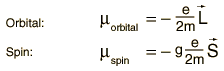.
where g is the spin g-factor and has a value of about 2, implying that the spin angular momentum is twice as effective in producing a magnetic moment.

The interaction energy of an atomic electron can then be written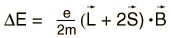.

which when evaluated in terms of the relevant quantum numbers takes the form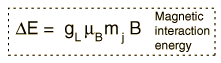.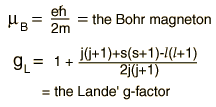### Show evaluation process

 Zeeman interaction Anomalous Zeeman effect
Index

Schrodinger equation concepts

Hydrogen concepts

 HyperPhysics***** Quantum Physics R Nave
Go Back

# Evaluating the Lande' g-Factor

The Lande' g-factor is a geometric factor which arises in the evaluation of the magnetic interaction which gives the Zeeman effect. The magnetic interaction energywhich is continuous in the classical case takes on the quantum formwhich is like a vector operation based on the vector model of angular momentum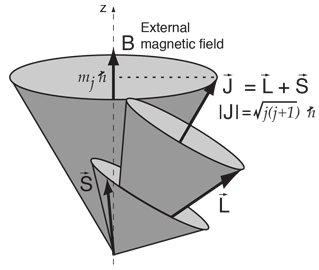The problem with evaluating this scalar product is that L and S continually change in direction as shown in the vector model. The strategy for dealing with this problem is to use the direction of the total angular momentum J as a coordinate axis and obtain the projection of each of the vectors in that direction. This is done by taking the scalar product of each vector with a unit vector in the J direction.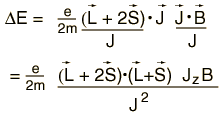These vector relationships must be evaluated and expressed in terms of quantum numbers in order to evaluate the energy shifts. Carrying out the scalar products above leads to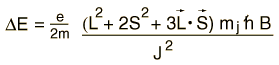Show reduction to standard form
Index

Schrodinger equation concepts

 HyperPhysics***** Quantum Physics R Nave
Go Back

# Lande' g-Factor Evaluation

 In the evaluation of the Lande' g-factor by the vector model, the expressionmust be simplified. Evaluating the remaining scalar product requires the vector model for total angular momentum.The law of cosines can be employed for evaluation of the scalar product.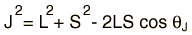Expressing this in terms of the external angle gives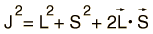Rearranging gives the scalar product term needed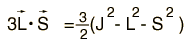Substitution into the energy expression gives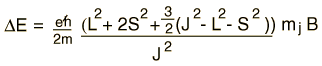which can be reduced to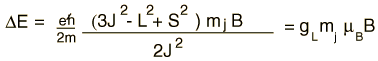where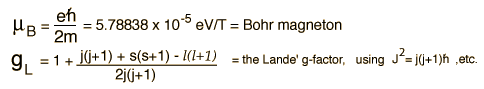Discussion of magnetic interaction Anomalous Zeeman effect
Index

Schrodinger equation concepts

 HyperPhysics***** Quantum Physics R Nave
Go Back